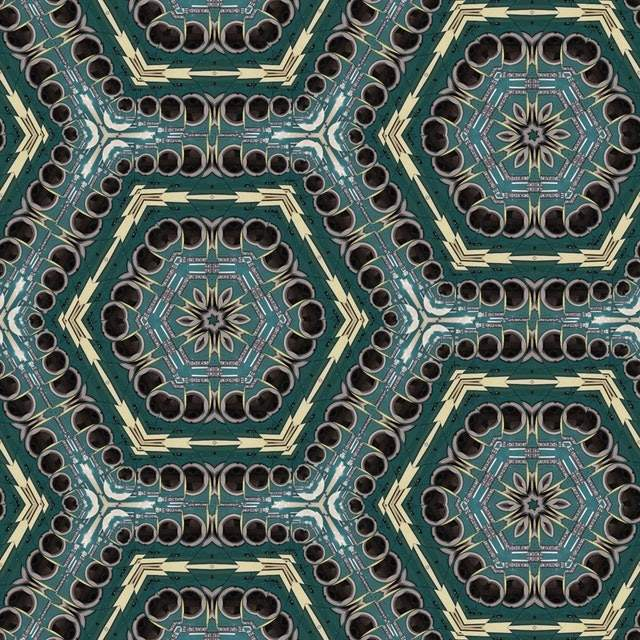EO as Exercises of Open/closed principle

By | March 6, 2020Spis treści

Open/closed principle

All classes should be open for enlargement and closed for modifications.How to use OCP in practice?

You should use interfaces and abstraction, not to become dependent on implementation details.

Difficulties in using OCP

Sometimes is hard to decide, what is implementing detail and in which direction the application is going.

Example

To exercises OCP I took a simple example. We have Calculator, which calculates figure area.In first version I am using switch, to recognise a specific figure.

class AreaCalculator
{
public AreaCalculator(List<object> figures)
{
this.figures = figures;
}
public double Calculate()
{
double area = 0;
foreach (var figure in figures)
{
switch (figure)
{
case Square square:
area += square.GetWidth() * square.GetWidth();
break;
case Rectangle rectangle:
area += rectangle.GetWidth() * rectangle.Getheight();
break;
case Circle circle:
area += Math.PI * Math.Pow(circle.GetRadius(), 2);
break;
default:
area += area;
break;
}
}
return area;
}
}

class Square
{
public Square(double width)
{
this.width = width;
}
internal double GetWidth()
{
return width;
}
}
class Rectangle
{

public Rectangle(double width, double height)
{
this.width = width;
this.height = height;
}
internal double GetWidth()
{
return width;
}
internal double Getheight()
{
return height;
}
}
class Circle
{
{
}
{
}
}
class Program
{
static void Main(string[] args)
{
List<object> figures = new List<object>()
{
new Square(5),

};
AreaCalculator areaCalculator = new AreaCalculator(figures);

Debug.Assert(areaCalculator.Calculate() == 25);

List<object> figures2 = new List<object>()
{
new Square(5),
new Rectangle(5,3),

};
Debug.Assert(new AreaCalculator(figures2).Calculate() == (25 + 15));
List<object> figures3 = new List<object>()
{
new Square(5),
new Rectangle(5,3),
new Circle(5),

};
Debug.Assert(new AreaCalculator(figures3).Calculate()
== (25 + 15 + Math.PI * 25));
}
}

After refactoring, I have figure classes, which implement the interface to calculate figure area. The calculator only sums all figure areas.

class AreaCalculator
{
public AreaCalculator(IEnumerable<IFigureAreaCalculator> figures)
{
this.figures = figures;
}

public double Calculate()
{
return figures.Sum(x => x.GetArea());
}
}
interface IFigureAreaCalculator
{
double GetArea();
}
class Square : IFigureAreaCalculator
{
public Square(double width)
{
this.width = width;
}

public double GetArea()
{
return width * width;
}

internal double GetWidth()
{
return width;
}
}
class Rectangle : IFigureAreaCalculator
{

public Rectangle(double width, double height)
{
this.width = width;
this.height = height;
}
internal double GetWidth()
{
return width;
}
internal double Getheight()
{
return height;
}

public double GetArea()
{
return width * height;
}
}
class Circle : IFigureAreaCalculator
{
{
}

public double GetArea()
{
}

{
}
}
class Program
{
static void Main(string[] args)
{
var figures = new List<IFigureAreaCalculator>()
{
new Square(5),

};
AreaCalculator areaCalculator = new AreaCalculator(figures);

Debug.Assert(areaCalculator.Calculate() == 25);

var figures2 = new List<IFigureAreaCalculator>()
{
new Square(5),
new Rectangle(5,3),

};
Debug.Assert(new AreaCalculator(figures2).Calculate() == (25 + 15));

var figures3 = new List<IFigureAreaCalculator>()
{
new Square(5),
new Rectangle(5,3),
new Circle(5),

};
Debug.Assert(new AreaCalculator(figures3).Calculate() == (25 + 15 + Math.PI * 25));
}
}

Adding a new figure does not require any changes in the AreaCalculator.